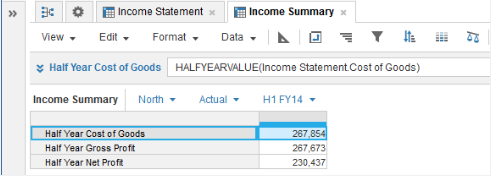1. Calculation functions
2. All Functions
3. Time and Date Functions
4. HALFYEARVALUEReturns the half-year value of the source line item or property that matches the time summary method set for the source.

## Syntax

`HALFYEARVALUE(x)`

where:

• x Line item - number, Boolean, date, time period, list, text.

## Format

Input Format Output Format

x: Line item - number, Boolean, date, time period, list, text.

Matches the input format.

## Arguments

The function uses the following arguments:

• x: Number, Boolean, date, time period, list item, text.

## Constraints

The function has the following constraints:

• The result formatting must match the source formatting.
• The result must have a timescale applied.
• Half-Year Totals must be selected in the model calendar.
• If the time summary method is None, only numbers get values. Use other time summary methods to get values for Booleans, dates, time periods, list items, and text. See Summary Methods and Time Aggregation for more information.

## Examples

### Sum example

In this example, an Income Statement source module shows gross and net profit by region for actual and forecast versions: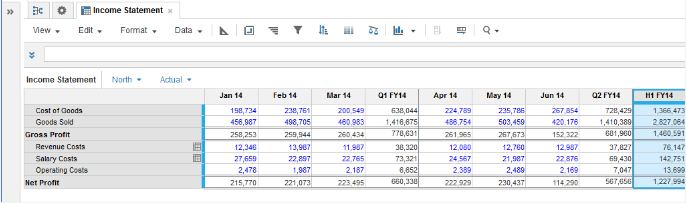The time summary method is set to Sum for the Cost of Goods, Gross Profit, and Net Profit line items:You can use HALFYEARVALUE in result line items in an Income Summary module that has a timescale applied to pull in any required half-year totals:

`HALFYEARVALUE(Income Statement.Cost of Goods)`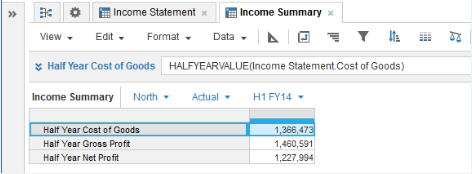Ensure that the time summary method you select for the result line items gives you the values that you want.

```HALFYEARVALUE(Income Statement.Cost of Goods)
```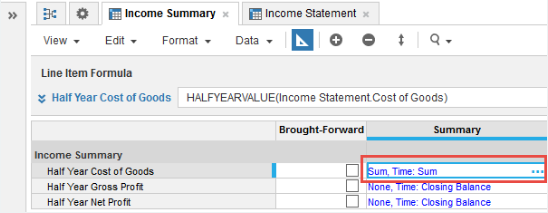In this example, If you change the time summary method for the Half Year Cost of Goods result line item from Closing Balance to Sum, the value returned for the H1 FY14 selection, where the timescale for the result line item is set to months, is incorrect. The value returned is the multiple of each month's half-year values:

6 x 1,366,473 = 8,198,838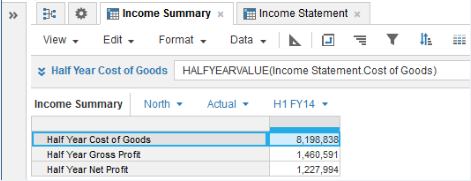### Max example

Take the first example and change the time summary method for the source line items to Max: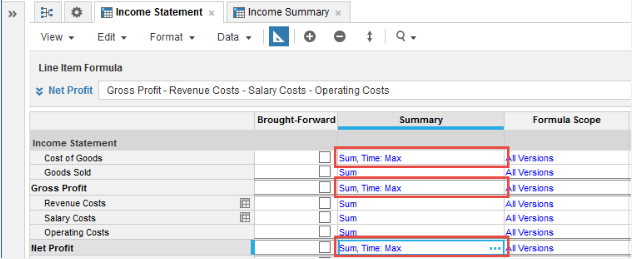The result line items in the Income Summary module adjust to show the maximum month value for the selected half-year:

`HALFYEARVALUE(Income Statement.Cost of Goods)`